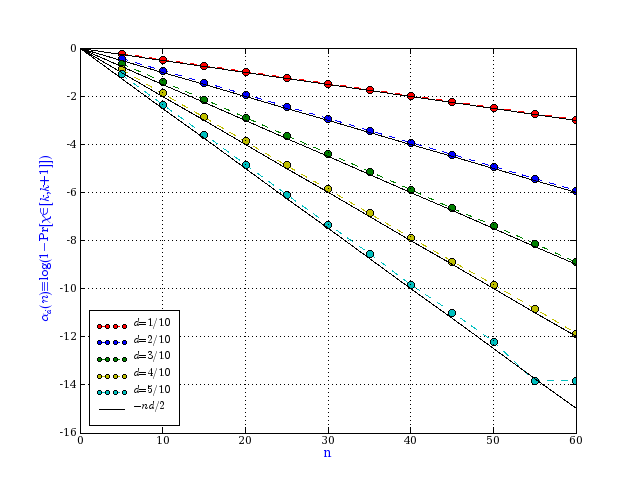Keith Briggshome · papers · thesis · talks · meetings · records · maths notes « · software · languages · music · travel · cv · students · maps · place-names · people · photos · links · ex libris · site map### Graph theory and Lambert's W function

The purpose of these notes is to point out two lesser-known applications of Lambert's W function occurring implicitly in two areas of graph theory.

Lambert's W function is defined as the inverse of the map w→w*exp(w) on the interval (-1/e,∞). I use the principal branch, defined as the greater value if there are two inverses (which happens on (-1/e,0)). See the mathworld article for more details. See here for programs to compute W.

Recall the well-known result concerning rooted labelled trees: -W(-z) has a Taylor expansion with coefficients nn-1/n!, in which the term nn-1 enumerates rooted labelled trees on n nodes. This result is combinatorial; by contrast the two applications of W below are analytic. Below, G(n,m) is the random graph model in which there are n nodes and m edges distributed uniformly and independently, and G{n,p} is the random graph model in which there are n nodes and each possible edge appears independently with probability p.

#### The random graph phase transition

See B. Bollabás, Modern graph theory, page 241. Bollabás shows that in the random graph G(n,cn/2) on n nodes, with c>1, the relative size L(1)/n of the largest component satisfies |L(1)/n - γ| < ω/sqrt(n) for almost every graph (as n→∞), where γ is given as the solution of e-cγ=1-γ. Here ω is the size of the largest clique.

In fact, this means that γ is given by γ(c)=1+W(-c/ec)/c.

Here is a simulation to verify this. For each value of c, I generated 1000 random graphs of 10,000 nodes, and computed the size of the largest component (red dots). The green line is given by γ(c). This is the famous `birth of the giant component'. Note the larger variance near c=1, a typical phase transition behaviour.Note that for c near 1, we have γ(c)=2(c-1)-8(c-1)2/3+...; and for c near ∞, we have γ(c)=1-exp(-c)-c*exp(-2c)+.... The next plot confirms this large c behaviour.#### The two possible values of the chromatic number

See D. Achlioptas & A. Naor, The two possible values of the chromatic number of a random graph Annals of Mathematics, 162 (2005) (available here). The authors show that for fixed d with probability 1 as n→∞, the chromatic number of G{n,d/n} is either k or k+1, where k is the smallest integer such that d < 2klog(k).

In fact, this means that k is given by ⌈d/(2W(d/2))⌉.

Here is a simulation to verify this. For each value of d, I generated 10 random graphs each of 200 nodes, and computed the exact chromatic number (red dots). The green lines are given by d/(2W(d/2))+1/2 and d/(2W(d/2))+3/2.We can go further and look at the rate of approach to probability 1. The next graph (each point is the average of 1 million trials) suggests that for small d, we have Prob[χ ∈ [k,k+1]] ≈ 1-exp(-dn/2). As far as I know, this conjecture is unproven.This website uses no cookies. This page was last modified 2013-05-12 10:17 by.